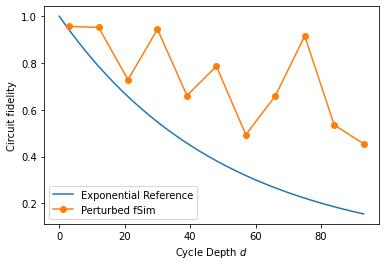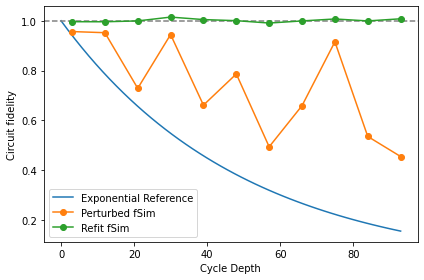# XEB and Coherent Error

try:
import cirq
except ImportError:
print("installing cirq...")
!pip install --quiet cirq
print("installed cirq.")

import numpy as np

import cirq
from cirq.contrib.svg import SVGCircuit


## Set up Random Circuits

We create a set of 10 random, two-qubit circuits which uses SINGLE_QUBIT_GATES to randomize the circuit and SQRT_ISWAP as the entangling gate. We will ultimately truncate each of these circuits according to cycle_depths. Please see the XEB Theory notebook for more details.

exponents = np.linspace(0, 7/4, 8)
exponents

array([0.  , 0.25, 0.5 , 0.75, 1.  , 1.25, 1.5 , 1.75])

import itertools
SINGLE_QUBIT_GATES = [
cirq.PhasedXZGate(x_exponent=0.5, z_exponent=z, axis_phase_exponent=a)
for a, z in itertools.product(exponents, repeat=2)
]
SINGLE_QUBIT_GATES[:10], '...'

([cirq.PhasedXZGate(axis_phase_exponent=0.0, x_exponent=0.5, z_exponent=0.0),
cirq.PhasedXZGate(axis_phase_exponent=0.0, x_exponent=0.5, z_exponent=0.25),
cirq.PhasedXZGate(axis_phase_exponent=0.0, x_exponent=0.5, z_exponent=0.5),
cirq.PhasedXZGate(axis_phase_exponent=0.0, x_exponent=0.5, z_exponent=0.75),
cirq.PhasedXZGate(axis_phase_exponent=0.0, x_exponent=0.5, z_exponent=1.0),
cirq.PhasedXZGate(axis_phase_exponent=0.0, x_exponent=0.5, z_exponent=1.25),
cirq.PhasedXZGate(axis_phase_exponent=0.0, x_exponent=0.5, z_exponent=1.5),
cirq.PhasedXZGate(axis_phase_exponent=0.0, x_exponent=0.5, z_exponent=1.75),
cirq.PhasedXZGate(axis_phase_exponent=0.25, x_exponent=0.5, z_exponent=0.0),
cirq.PhasedXZGate(axis_phase_exponent=0.25, x_exponent=0.5, z_exponent=0.25)],
'...')

import cirq_google as cg
from cirq.experiments import random_quantum_circuit_generation as rqcg

q0, q1 = cirq.LineQubit.range(2)

# Make long circuits (which we will truncate)
n_circuits = 10
circuits = [
rqcg.random_rotations_between_two_qubit_circuit(
q0, q1,
depth=100,
two_qubit_op_factory=lambda a, b, _: cirq.SQRT_ISWAP(a, b),
single_qubit_gates=SINGLE_QUBIT_GATES)
for _ in range(n_circuits)
]

# We will truncate to these lengths
max_depth = 100
cycle_depths = np.arange(3, max_depth, 9)
cycle_depths

array([ 3, 12, 21, 30, 39, 48, 57, 66, 75, 84, 93])


## Emulate coherent error

We request a $$\sqrt{i\mathrm{SWAP} }$$ gate, but the quantum hardware may execute something subtly different. Therefore, we move to a more general 5-parameter two qubit gate, cirq.PhasedFSimGate.

This is the general excitation-preserving two-qubit gate, and the unitary matrix of PhasedFSimGate(θ, ζ, χ, γ, φ) is:

[[1,                       0,                       0,            0],
[0,    exp(-iγ - iζ) cos(θ), -i exp(-iγ + iχ) sin(θ),            0],
[0, -i exp(-iγ - iχ) sin(θ),    exp(-iγ + iζ) cos(θ),            0],
[0,                       0,                       0, exp(-2iγ-iφ)]].


This parametrization follows eq (18) in https://arxiv.org/abs/2010.07965. Please read the docstring for cirq.PhasedFSimGate for more information.

With the following code, we show how SQRT_ISWAP can be written as a specific cirq.PhasedFSimGate.

sqrt_iswap_as_phased_fsim = cirq.PhasedFSimGate.from_fsim_rz(
theta=-np.pi/4, phi=0,
rz_angles_before=(0,0), rz_angles_after=(0,0))
np.testing.assert_allclose(
cirq.unitary(sqrt_iswap_as_phased_fsim),
cirq.unitary(cirq.SQRT_ISWAP),
atol=1e-8
)


We'll also create a perturbed version. Note the $$\pi/16$$ phi angle:

perturbed_sqrt_iswap = cirq.PhasedFSimGate.from_fsim_rz(theta=-np.pi/4, phi=np.pi/16,
rz_angles_before=(0,0), rz_angles_after=(0,0))
np.round(cirq.unitary(perturbed_sqrt_iswap), 3)

array([[1.   +0.j   , 0.   +0.j   , 0.   +0.j   , 0.   +0.j   ],
[0.   +0.j   , 0.707+0.j   , 0.   +0.707j, 0.   +0.j   ],
[0.   +0.j   , 0.   +0.707j, 0.707+0.j   , 0.   +0.j   ],
[0.   +0.j   , 0.   +0.j   , 0.   +0.j   , 0.981-0.195j]])


We'll use this perturbed gate along with the GateSubstitutionNoiseModel to create simulator which has a constant coherent error. Namely, each SQRT_ISWAP will be substituted for our perturbed version.

def _sub_iswap(op):
if op.gate == cirq.SQRT_ISWAP:
return perturbed_sqrt_iswap.on(*op.qubits)
return op

noise = cirq.devices.noise_model.GateSubstitutionNoiseModel(_sub_iswap)
noisy_sim = cirq.DensityMatrixSimulator(noise=noise)


## Run the benchmark circuits

We use the function sample_2q_xeb_circuits to execute all of our circuits at the requested cycle_depths.

from cirq.experiments.xeb_sampling import sample_2q_xeb_circuits
sampled_df = sample_2q_xeb_circuits(sampler=noisy_sim, circuits=circuits,
cycle_depths=cycle_depths, repetitions=10_000)

100%|██████████| 117/117 [00:12<00:00,  9.59it/s]


## Compute fidelity assuming SQRT_ISWAP

In contrast to the XEB Theory notebook, here we only have added coherent error (not depolarizing). Nevertheless, the random, scrambling nature of the circuits shows circuit fidelity decaying with depth (at least when we assume that we were trying to use a pure SQRT_ISWAP gate)

from cirq.experiments.xeb_fitting import benchmark_2q_xeb_fidelities
fids = benchmark_2q_xeb_fidelities(sampled_df, circuits, cycle_depths)

%matplotlib inline
from matplotlib import pyplot as plt

xx = np.linspace(0, fids['cycle_depth'].max())
plt.plot(xx, (1-5e-3)**(4*xx), label=r'Exponential Reference')

plt.plot(fids['cycle_depth'], fids['fidelity'], 'o-', label='Perturbed fSim')

plt.ylabel('Circuit fidelity')
plt.xlabel('Cycle Depth $d$')
plt.legend(loc='best')

<matplotlib.legend.Legend at 0x7fe21babc040>## Optimize PhasedFSimGate parameters

We know what circuits we requested, and in this simulated example, we know what coherent error has happened. But in a real experiment, there is likely unknown coherent error that you would like to characterize. Therefore, we make the five angles in PhasedFSimGate free parameters and use a classical optimizer to find which set of parameters best describes the data we collected from the noisy simulator (or device, if this was a real experiment).

fids_opt = simulate_2q_xeb_fids(sampled_df, pcircuits, cycle_depths, param_resolver={'theta': -np.pi/4, 'phi': 0.1})

import multiprocessing
pool = multiprocessing.get_context('spawn').Pool()

from cirq.experiments.xeb_fitting import \
parameterize_circuit, characterize_phased_fsim_parameters_with_xeb, SqrtISwapXEBOptions

options = SqrtISwapXEBOptions(
characterize_theta=True,
characterize_phi=True,
characterize_chi=False,
characterize_gamma=False,
characterize_zeta=False
)
p_circuits = [parameterize_circuit(circuit, options) for circuit in circuits]
res = characterize_phased_fsim_parameters_with_xeb(
sampled_df,
p_circuits,
cycle_depths,
options,
pool=pool,
xatol=1e-3,
fatol=1e-3)

Simulating with theta =  -0.785 phi   =       0
Loss:   0.265
Simulating with theta =  -0.685 phi   =       0
Loss:   0.607
Simulating with theta =  -0.785 phi   =     0.1
Loss:  0.0794
Simulating with theta =  -0.885 phi   =     0.1
Loss:   0.251
Simulating with theta =  -0.885 phi   =     0.2
Loss:   0.277
Simulating with theta =   -0.81 phi   =    0.05
Loss:   0.133
Simulating with theta =   -0.71 phi   =    0.05
Loss:     0.4
Simulating with theta =  -0.842 phi   =  0.0875
Loss:   0.119
Simulating with theta =  -0.817 phi   =   0.138
Loss:  0.0326
Simulating with theta =   -0.82 phi   =   0.181
Loss:  0.0302
Simulating with theta =  -0.764 phi   =   0.194
Loss:  0.0166
Simulating with theta =  -0.724 phi   =   0.247
Loss:  0.0695
Simulating with theta =  -0.798 phi   =   0.275
Loss:  0.0589
Simulating with theta =  -0.795 phi   =   0.231
Loss:  0.0111
Simulating with theta =  -0.739 phi   =   0.244
Loss:  0.0359
Simulating with theta =  -0.799 phi   =   0.197
Loss: 0.00333
Simulating with theta =  -0.831 phi   =   0.234
Loss:  0.0834
Simulating with theta =   -0.78 phi   =   0.204
Loss: -0.000941
Simulating with theta =  -0.785 phi   =    0.17
Loss: 0.00865
Simulating with theta =  -0.787 phi   =   0.185
Loss: 0.00101
Simulating with theta =  -0.768 phi   =   0.192
Loss:  0.0115
Simulating with theta =  -0.792 phi   =   0.196
Loss: -0.000596
Simulating with theta =  -0.785 phi   =   0.215
Loss: -0.000775
Simulating with theta =  -0.773 phi   =   0.223
Loss: 0.000963
Simulating with theta =  -0.787 phi   =   0.202
Loss: -0.00146
Simulating with theta =  -0.783 phi   =   0.192
Loss: 0.000499
Simulating with theta =  -0.784 phi   =   0.209
Loss: -0.00146
Simulating with theta =  -0.791 phi   =   0.207
Loss: -0.000215
Simulating with theta =  -0.783 phi   =   0.205
Loss: -0.00147
Simulating with theta =  -0.786 phi   =   0.198
Loss: -0.00129
Simulating with theta =  -0.785 phi   =   0.206
Loss: -0.00155
Simulating with theta =   -0.78 phi   =   0.209
Loss: -0.00124
Simulating with theta =  -0.785 phi   =   0.204
Loss: -0.00155
Simulating with theta =  -0.787 phi   =   0.205
Loss: -0.00139
Simulating with theta =  -0.784 phi   =   0.205
Loss: -0.00155
Simulating with theta =  -0.786 phi   =   0.205
Loss: -0.00151
Simulating with theta =  -0.784 phi   =   0.205
Loss: -0.00156
Simulating with theta =  -0.785 phi   =   0.203
Loss: -0.00153
Simulating with theta =  -0.785 phi   =   0.205
Loss: -0.00156
Simulating with theta =  -0.784 phi   =   0.206
Loss: -0.00154
Simulating with theta =  -0.785 phi   =   0.205
Loss: -0.00156

xx = np.linspace(0, fids['cycle_depth'].max())
p_depol = 5e-3 # from above
plt.plot(xx, (1-p_depol)**(4*xx), label=r'Exponential Reference')
plt.axhline(1, color='grey', ls='--')

plt.plot(fids['cycle_depth'], fids['fidelity'], 'o-', label='Perturbed fSim')
plt.plot(res.fidelities_df['cycle_depth'], res.fidelities_df['fidelity'], 'o-', label='Refit fSim')

plt.ylabel('Circuit fidelity')
plt.xlabel('Cycle Depth')
plt.legend(loc='best')
plt.tight_layout()[{ "type": "thumb-down", "id": "missingTheInformationINeed", "label":"Missing the information I need" },{ "type": "thumb-down", "id": "tooComplicatedTooManySteps", "label":"Too complicated / too many steps" },{ "type": "thumb-down", "id": "outOfDate", "label":"Out of date" },{ "type": "thumb-down", "id": "samplesCodeIssue", "label":"Samples / code issue" },{ "type": "thumb-down", "id": "otherDown", "label":"Other" }]
[{ "type": "thumb-up", "id": "easyToUnderstand", "label":"Easy to understand" },{ "type": "thumb-up", "id": "solvedMyProblem", "label":"Solved my problem" },{ "type": "thumb-up", "id": "otherUp", "label":"Other" }]